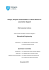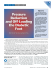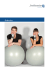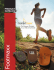# THE PRESENT USE OF THE UCBL FOOT ORTHOSIS

## Transcription

THE PRESENT USE OF THE UCBL FOOT ORTHOSIS
```THE PRESENT USE OF THE
UCBL FOOT ORTHOSIS
Michael J. Quigley, C . P . O .
1
In A u g u s t 1971, the C o m m i t t e e o n P r o s t h e t i c s
R e s e a r c h and D e v e l o p m e n t ( C P R D ) of the N a ­
tional R e s e a r c h Council c o m p l e t e d a n e v a l u a t i o n
of four
VAPC
lower-limb
Single-Bar
orthoses. They
were
Knee-Ankle-Foot
the
Orthosis
( K A F O ) . the U C B L Dual Axis A n k l e - F o o t Or­
thosis ( A F O ) , the N e w York University
insert
Ankle-Foot Orthosis, and the U C B L Shoe Insert
F o o t O r t h o s i s ( F O ) (Figs. 1 a n d 2). T h e latter t w o
o r t h o s e s w e r e c o n s i d e r e d to be valuable
tions to patient services, and
it w a s
recom­
m e n d e d t h a t t h e y b e i n c l u d e d in o r t h o t i c s e d u c a ­
tion p r o g r a m s (2).
Fig. 2. Principles of the U C B L foot o r t h o s i s applied to
the foot section of a p o l y p r o p y l e n e ankle-foot o r t h o s i s .
To determine
the a c c e p t a n c e
of the
UCBL
s h o e insert foot o r t h o s i s , h o w the t e c h n i q u e w a s
l e a r n e d , a n d s o m e t h i n g a b o u t t h e e x p e r i e n c e s in
the field,
Thirty-five
a
limited
certified
survey
was
conducted.
orthotics
and
prosthetics-
o r t h o t i c s facilities w e r e s e l e c t e d r a n d o m l y
the
1974 Registry
of Accredited
Facilities
from
(1).
T h i s r e p r e s e n t e d a n equal d i s t r i b u t i o n of p r a c ­
titioners from t w e n t y cities. T h e questionnaires
( A p p e n d i x A) c o n s i s t e d of a section to be c o m ­
p l e t e d by the p h y s i c i a n a n d a s e c t i o n to be c o m ­
Fig. 1. The U C B L foot o r t h o s i s .
pleted
quested
1Staff P r o s t h e t i s t / O r t h o t i s t . C o m m i t t e e on Prosthet­
ics R e s e a r c h and D e v e l o p m e n t . National A c a d e m y of
S c i e n c e s , W a s h i n g t o n , D. C. 20418.
by the orthotist. T h e orthotist w a s
to forward
the questionnaire
to
p h y s i c i a n after his s e c t i o n w a s c o m p l e t e d .
re­
the
The
q u e s t i o n n a i r e s w e r e s e n t o u t in J a n u a r y 1974. B y
M a r c h 1974. t w e n t y - n i n e of the f o r m s w e r e re-
t u r n e d . T h e following information w a s t a k e n
from these forms.
T h e U C B L foot orthosis w a s used by 75 per­
cent of the surveyed practitioners. H o w e v e r ,
only seven of the t w e n t y - o n e practitioners w h o
use this orthosis d o so regularly. Of the remaining
fourteen r e s p o n d e n t s , five use t h e orthosis only
w h e n it is requested by a certain physician, five
rarely use it, two use it for special conditions
only, and one stated he uses the orthosis only as a
last resort.
An a t t e m p t w a s made to d e t e r m i n e how the
practitioners were m a d e a w a r e of the U C B L foot
o r t h o s i s . T h e literature rated as the most c o m ­
m o n s o u r c e of i n f o r m a t i o n . A r t i c l e s o n t h e
U C B L foot orthosis a p p e a r e d in the Bulletin of
Prosthetics Research (3) in S e p t e m b e r 1969 and
in Orthotics and Prosthetics (4)in March 1972. In
a d d i t i o n . N e w Y o r k U n i v e r s i t y published an
evaluation report (5) on the orthosis in 1969. T h e
prosthetics-orthotics education c o u r s e s were the
second most c o m m o n source of information on
the subject. Word of m o u t h rated as the third
main channel of c o m m u n i c a t i o n s , since s o m e re­
s p o n d e n t s stated that orthotists, podiatrists, e t c . ,
had informed t h e m of the t e c h n i q u e .
PRESCRIPTION CONSIDERATION
Eight areas considered to influence the pre­
scription and use of the U C B L foot orthosis w e r e
c o v e r e d in the q u e s t i o n n a i r e . T h e most c o m m o n
prescription was bilateral o r t h o s e s for a patient
25-40 years old with pes planus.
T h e pathologies most c o m m o n l y treated with
the U C B L foot orthosis are pes planus and ar­
thritis. Following t h e s e , in o r d e r of frequency,
are plantar fasciitis, metatarsalgia, polio, cere­
bral palsy and peroneal palsy.
R e s p o n d e n t s indicated that the orthosis is used
equally on males and females and is fitted bilater­
ally the vast majority of the time. T h e age groups
that use the U C B L foot orthosis most often are
between 1-12 years of age and b e t w e e n 25-40
years of age.
T h e major disadvantage of using this orthosis is
the e x p e n s e , a fact that was underscored by half
of t h e r e s p o n d e n t s . T h e o t h e r d i s a d v a n t a g e s
c h e c k e d off by the practitioners are that a wider
shoe is s o m e t i m e s n e e d e d , that the orthosis slips
up and d o w n in the shoe, and that breakage oc­
c u r s . T w o practitioners felt the orthosis is dif­
ficult to fit.
T h e major a d v a n t a g e s of using this orthosis is
that is provides proper foot support, allows the
patient to change s h o e s , eliminates shoe modifi­
cations and the need for o r t h o p e d i c s h o e s . T h e
practitioners also felt that the U C B L foot or­
thosis provides i m p r o v e d c o s m e s i s and gives
consistent relief from pain.
Most of the r e s p o n d e n t s indicated that the or­
thosis usually lasts longer t h a n a year before re­
placement is n e c e s s a r y , although a few prac­
titioners stated that it only lasts up to one year.
T h e major reason that the U C B L foot orthosis
n e e d s r e p l a c e m e n t is a loss of fit with t i m e .
Breakage is the second most c o m m o n reason re­
placement w a s needed. O n e practitioner stated
that he replaces the o r t h o s e s in cases when he
wants to increase progressively the a m o u n t of
foot c o r r e c t i o n .
CASTING, FABRICATION AND FITTING
This part of the survey w a s structured to de­
termine if the original t e c h n i q u e is still practiced,
w h a t materials and m e t h o d s are presently used
for fabrication, fitting p r o b l e m s e n c o u n t e r e d and
solutions to these p r o b l e m s .
Nearly e v e r y practitioner stated that he uses
the s a m e m e t h o d of wrapping to obtain the mold
as was d e s c r i b e d originally in the l i t e r a t u r e .
Manual alignment of t h e foot and ankle is prac­
ticed by all r e s p o n d e n t s , as is the use of the
c o n t o u r e d casting b o a r d s for positioning the pa­
tient. O n e - q u a r t e r of the orthotists use standard
plaster b a n d a g e rather than the elastic type origi­
nally r e c o m m e n d e d , and one-third of the or­
thotists no longer use the balloon method for
casting.
Polyester resin is used exclusively by eight of
the o r t h o t i s t s , four use both polyester resin and
p o l y p r o p y l e n e , five use p o l y p r o p y l e n e exclu­
sively, one uses polyethylene and one uses acryl­
ic.
It is interesting that of the seven practitioners
that had breakage p r o b l e m s , six use polyester
resin for fabrication and o n e uses polyethylene.
N o n e of the orthotists that utilize polypropylene
exclusively mentioned breakage p r o b l e m s .
T h e most c o m m o n fitting problem is pain at the
location of the navicular (scaphoid) b o n e , which
is located medially at the apex of the arch of t h e
foot. Shoes being too tight w h e n the orthosis is
w o r n is the next most c o m m o n problem, followed
by pistoning of the foot in the s h o e , and difficul-
ties in establishing t h e trimline at t h e metatarsal
area. O n e practitioner stated that he has his pa­
tients acquire a pair of shoes that will a c c o m m o ­
date t h e o r t h o s i s .
N o n e of t h e orthotists d o a n y shoe modifica­
tions in addition to using the U C B L foot o r t h o s i s .
Six orthotists consistently modify t h e foot or­
thosis. Wedges and metatarsal relief pads are
added by t w o practitioners. O n e orthotist uses
S p e n c o * buildups, p r e s u m a b l y for better weight
d i s t r i b u t i o n a n d r e d u c t i o n of s h e a r s t r e s s e s .
A n o t h e r adds a Velcro s t r a p o v e r t h e d o r s u m of
the foot t o prevent t h e foot from pistoning. T o
d e c r e a s e t h e sliding of t h e foot orthosis o n the
insole of the s h o e , o n e o r t h o t i s t lines the b o t t o m
surface of the orthosis with moleskin or thin
non-skid rubber.
DISCUSSION
T h e U C B L foot orthosis w a s first publicized in
S e p t e m b e r 1969, and introduced in t h e education
programs in late 1971. T h e fact that 75 p e r c e n t of
the s u r v e y e d practitioners had used t h e orthosis
by J a n u a r y 1974 is a t e s t i m o n y to t h e speed t h a t
p r o v e n r e s e a r c h in this field is applied to t h e
*A foam rubber that is impregnated with nitrogen
bubbles.
patient. P r o b a b l y n o o t h e r medical o r paramedi­
cal specialty c a n realize these patient benefits
from r e s e a r c h only four and one-half years after
the initial introduction of a t e c h n i q u e .
T h e utilization of this orthosis m a y d e c r e a s e in
the future as t h e r m o p l a s t i c , t h e r m o f o r m e d
lower-limb o r t h o s e s gain a c c e p t a n c e . H o w e v e r ,
the U C B L foot alignment principles still apply t o
, the foot section of thermoplastic ankle-foot o r t h o ­
ses and knee-ankle-foot o r t h o s e s , and should be
used w h e n e v e r possible.
REFERENCES
1. American Board for Certification in Orthotics &
Prosthetics, Inc., 1974 Registry of Accredited
and Certified Individuals in Orthotics and
Facilities
Prosthetics,
Washington, D.C., 1974.
2. Committee on Prosthetics Research and De­
velopment, A Clinical Evaluation
of Four
Lower-Limb
Orthoses, National Academy of Sciences, Washington,
D.C., 1971.
3. Henderson, W . H . , and J. W. Campbell, UC-BL
shoe insert: casting and fabrication. Bull. Pros. Res.
10-11:215-235, Spring 1969.
4. LeBlanc, Maurice A., Aclinical evaluation offour
lower-limb orthoses. Orth. and Pros. 26:1:27-47,
March 1972.
5. Mereday, C., C.M.E. Dolan, and R. Lusskin,
Evaluation
Planus.
of the UCBL Shoe Insert in "Flexible"
New York University, September 1969.
Pes
APPENDIX A
COMMITTEE ON PROSTHETICS RESEARCH AND DEVELOPMENT
DIVISION OF MEDICAL SCIENCES—NATIONAL RESEARCH COUNCIL
```

### Lower Extremity Orthoses: A Primer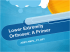### Eneslow Custom Department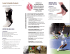### Untitled copy 2 - Danmar Cranial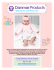### PDF Now - Foot Levelers Education Resource Center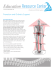### View as PDF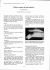### Foundations of Pediatric Orthotics - Academy of Pediatric Physical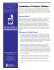### UCBL Orthosis - Presque Isle Medical Technologies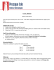### Training Program for the Tibialis Posterior Muscle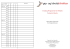### ToeOFF Family Professional Instructions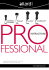### Inside perspective: the role of the podiatrist in diabetes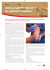### Dance Teacher Prompt Pages.indd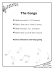### Essentials Pack - Foot Levelers Education Resource Center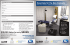### Athlete`s foot - BMJ Best Practice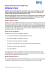### Dan Baker`s R2 Manual.indd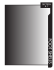### Should Functional Ambulation be A Goal for Paraplegic Persons?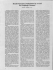### Product Catalogue - Supports and Orthoses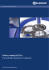### 2009 Alumni Newsletter - FOOT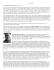### Product catalog Supports and Orthoses Motion is Life.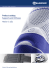### Design, Analysis and Simulation of a Novel Device for Locomotion